DraftKings Make Playoffs Odds
-4000
0.03 to 1Avalanche
5.8% implied probability

No changes have been recorded yet.
-1000
0.1 to 1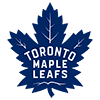Maple Leafs
5.4% implied probability

No changes have been recorded yet.
-900
0.11 to 1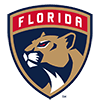Panthers
5.4% implied probability

-750
0.13 to 1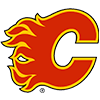Flames
5.3% implied probability

-750
0.13 to 1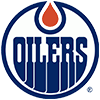Oilers
5.3% implied probability

-700
0.14 to 1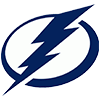Lightning
5.2% implied probability

No changes have been recorded yet.
-500
0.2 to 1Penguins
5.0% implied probability

No changes have been recorded yet.
-500
0.2 to 1Wild
5.0% implied probability

-500
0.2 to 1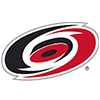Hurricanes
5.0% implied probability

-240
0.42 to 1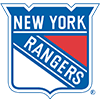Rangers
4.2% implied probability

-220
0.45 to 1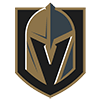Golden Knights
4.1% implied probability

-200
0.5 to 1Predators
4.0% implied probability

No changes have been recorded yet.
-190
0.53 to 1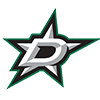Stars
3.9% implied probability

-190
0.53 to 1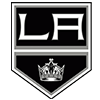Kings
3.9% implied probability

-190
0.53 to 1Capitals
3.9% implied probability

-190
0.53 to 1Bruins
3.9% implied probability

-170
0.59 to 1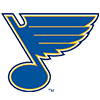Blues
3.8% implied probability

-130
0.77 to 1Canucks
3.4% implied probability

No changes have been recorded yet.
+100
1 to 1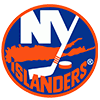Islanders
3.0% implied probability

+140
1.4 to 1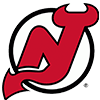Devils
2.5% implied probability

No changes have been recorded yet.
+180
1.8 to 1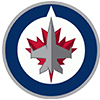Jets
2.1% implied probability

No changes have been recorded yet.
+200
2 to 1Senators
2.0% implied probability

+290
2.9 to 1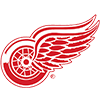Red Wings
1.5% implied probability

No changes have been recorded yet.
+300
3 to 1Kraken
1.5% implied probability

+500
5 to 1Ducks
1.0% implied probability

No changes have been recorded yet.
+600
6 to 1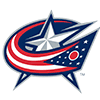Blue Jackets
0.9% implied probability

No changes have been recorded yet.
+600
6 to 1Sabres
0.9% implied probability

+900
9 to 1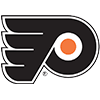Flyers
0.6% implied probability

+900
9 to 1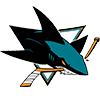Sharks
0.6% implied probability

No changes have been recorded yet.
+1500
15 to 1Canadiens
0.4% implied probability

No changes have been recorded yet.
+2500
25 to 1Coyotes
0.2% implied probability

+2500
25 to 1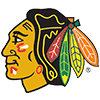Blackhawks
0.2% implied probability